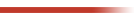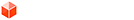## [Code] Parsing arithmetic formulas

### [Code] Parsing arithmetic formulas

Here are a set of standalone methods that can parse a string as an arithmetic value or formula for Integers, Floats and Lengths.

They are actually derived from some work I have done in LibFredo6 (in module Traductor).

I enclosed the methods in a module called ArithmeString, which you can of course change if you enclose some of this code in your script.
• string_to_integer --> parse a string as an integer
• string_to_integer_formula --> parse a string as a formula evaluating as an integer
• string_to_float --> parse a string as a float
• string_to_float_formula --> parse a string as a formula evaluating as a float
• string_to_length --> parse a string as a length
• string_to_length_formula --> parse a string as a formula evaluating as a length

Note that all methods accepts the "." or the "," as valid decimal delimiter, regardless of the underlying locale of the operating system and SU version language.

All the formula methods accepts parentheses to manage more complex calculations.
For lengths, there is a logical order to respect for specifying units and numbers (ex: 2cm * 4, NOT 4 * 2cm)

Arithmetic parsing.rb

Fredo
1fredo6### Re: [Code] Parsing arithmetic formulas

Thanks Fredo
I knew someone must have done more on it.
The nearest I have is in my roof tools where you can specify a slope in degrees [25.0], a ridge height [^3.5] or a pitch [2:3]. The tool then spots which type it is and runs the appropriate method, e.g. using a Math.tan() on the A:B version...
0
TIGTIG
Global Moderator

### Re: [Code] Parsing arithmetic formulas

TIG wrote:Thanks Fredo
I knew someone must have done more on it.
The nearest I have is in my roof tools where you can specify a slope in degrees [25.0], a ridge height [^3.5] or a pitch [2:3]. The tool then spots which type it is and runs the appropriate method, e.g. using a Math.tan() on the A:B version...

TIG,

Thanks. I wonder why this is not embedded in Ruby (or maybe it is in a package that is not included in the Ruby distribution of Sketchup). My real problem is with the length. I have real difficulty with the architectural notation!

Fredo
0fredo6### Re: [Code] Parsing arithmetic formulas

0
I'm not here much anymore. But a PM will fire email notifications.Dan Rathbun
PluginStore Author### Re: [Code] Parsing arithmetic formulas

Fredo6 wrote: I wonder why this is not embedded in Ruby (or maybe it is in a package that is not included in the Ruby distribution of Sketchup).

I checked the extended Ruby libraries, but nothing seems to be like this (or the Perl module or Java class, MathString.)

I also did a search at RubyForge to see if anyone else had a similar project, but did not find anything.

Fredo6 wrote:My real problem is with the length. I have real difficulty with the architectural notation!

The Sketchup API already extended the Ruby base String class with a .to_l method.
Can you use that method call within your module's length converter methods?

I also wonder if this module would be easier to use as a custom String subclass?
0
I'm not here much anymore. But a PM will fire email notifications.Dan Rathbun
PluginStore Author### Re: [Code] Parsing arithmetic formulas

I used this code in my joint tools and found it very useful as the user could enter equation if they do not know the actual value. However I could not make it work if the user entered the " for inches as sketch-up does.

Keith
0

ktkoh
PluginStore Author